## Week 3 Coding Lecture 2: PA = LU decomposition

We have two different methods of solving systems of equations: Forward/back substitution and Gaussian elimination. We just saw that, at least for large systems, forward/back substitution is vastly faster than Gaussian elimination. We would therefore prefer to use forward/back substitution for all of our problems. Unfortunately, forward/back substitution only work in special cases. If our system isn't lower/upper triangular, then we can't use this faster method. We have already seen several examples of non-triangular systems, so we know that we can't hope that all systems will be triangular in general. However, it is possible that we could write all systems in some simple form so that we didn't have to use the full Gaussian elimination method. In particular, suppose that we could always rewrite a system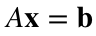in the form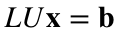,
where L is an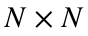lower triangular matrix and U is anupper triangular matrix. If this were true, it would be relatively easy to solve the system. To see how, note that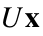is an (unknown)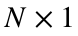vector (because it is the product of anmatrix U and anvector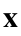). If we give this vector a new name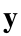, then we have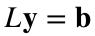,
where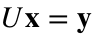.
Notice that the equationis easy to solve! L is a lower triangular matrix and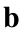is a known vector, so we can just use forward substitution, which takes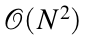flops. Once we do this, we know the vector, which means that we can solve. Again, this is easy to solve! Since U is upper triangular, we can just use back substitution, which also takesflops. We can therefore solve the original system in twosteps. Since big-oh notation ignores constant multiples, this is essentially the same as. This means that if we are given a system in the form, we can just use substitution twice instead of Gaussian elimination and therefore solve our system much faster.
Of course, it is unlikely that someone will simply hand you a system in this convenient form, so we need to find a method that calculates L and U from A. Through a somewhat lucky coincidence, it turns out that (almost) every matrix A can be written in this way, and that we can find L and U through Gaussian elimination. We will go through an example by hand and then turn to MATLAB.
Remember our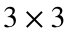system from earlier in the week:.
After performing the row operations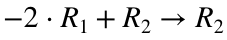,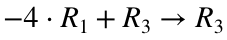,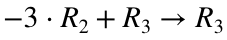,
we obtained the new system.
This new system is upper triangular, and we will use the resulting matrix as U. That is,.
The matrix L is somewhat more complicated, but we can create it by looking at the row operations we employed. L is always of the form,
where the entries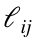are numbers that we have to determine. It turns out that these entries are just the coefficients we used in our row operations with the signs reversed. For instance, we used the row operationto zero out the 2nd row, 1st column of A, so the entry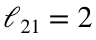(note that the sign has flipped). Likewise, we used the row operationto change the 3rd row, 1st column of A, so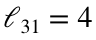. Finally, we used the row operationto change the 3rd row, 2nd column of A, so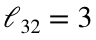. This means that.
We can use MATLAB to check that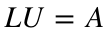:
b = [1; 1; -1];
A = [2 1 1; 4 3 3; 8 7 9]
A = 3×3
2 1 1 4 3 3 8 7 9
L = [1 0 0; 2 1 0; 4 3 1];
U = [2 1 1; 0 1 1; 0 0 2];
L*U
ans = 3×3
2 1 1 4 3 3 8 7 9
The process of finding L and U is called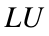-decomposition.
Once we have L and U, we can solve the original system with two steps of forward/back substitution. We first solve the equationwith forward substitution:
y = L\b;
Then we use that result to solvewith back substitution:
x = U\y
x = 3×1
1 0 -1
This is the same solution we found with Gaussian elimination originally.
A\b
ans = 3×1
1 0 -1

### Permutation matrices

We said above that almost every matrix could be written in the form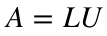. The "almost" is important, and it is related to the fact that Gaussian elimination does not always work. We established earlier in the week that Gaussian elimination could fail if there were a zero on the main diagonal of your matrix so that you couldn't continue eliminating coefficients. We also established that you could always solve this issue by reordering your equations.
MATLAB expresses "reordering equations" through something called a permutation matrix. A permutation matrix is just the identity matrix with some of the rows reordered. (Remember, the identity matrix is a square matrix with 1's on the diagonal and 0's everywhere else.) For instance,is a permutation matrix because it is theidentity matrix with the last row moved to the top.
If you multiply a permutation matrix by another matrix or vector, it just reorders the rows of the matrix/vector. For instance,
A
A = 3×3
2 1 1 4 3 3 8 7 9
P = [0 0 1; 1 0 0; 0 1 0];
P*A
ans = 3×3
8 7 9 2 1 1 4 3 3
That is,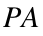is just A with the last row moved to the top.
If you have a system of equationsand you want to reorder the equations, you need to multiply both sides of the equation by P. So, for example, if we have the following A and b:
A
A = 3×3
2 1 1 4 3 3 8 7 9
b
b = 3×1
1 1 -1
then you could reorder the system by changing them toand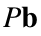:
P*A
ans = 3×3
8 7 9 2 1 1 4 3 3
P*b
ans = 3×1
-1 1 1
A matrix A can't always be written as, but if you reorder the rows of A first, then you can always write it in this form. In mathematical notation, this means that there is always a permutation matrix P, a lower triangular matrix L and an upper triangular matrix U such that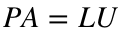.
We already saw how to compute L and U by hand. We won't worry about how to find P by hand, because it is somewhat more complicated and MATLAB will do it for us.
You can calculate these three matrices in MATLAB with the command lu. The syntax is as follows:
[L, U, P] = lu(A)
L = 3×3
1.0000 0 0 0.2500 1.0000 0 0.5000 0.6667 1.0000
U = 3×3
8.0000 7.0000 9.0000 0 -0.7500 -1.2500 0 0 -0.6667
P = 3×3
0 0 1 1 0 0 0 1 0
Notice that MATLAB did not find the same L and U we did. That is because we didn't reorder the rows of A, but MATLAB did. (You can tell by looking at P - it is not just the identity matrix.) We can confirm the relationship, though:
P*A
ans = 3×3
8 7 9 2 1 1 4 3 3
L*U
ans = 3×3
8 7 9 2 1 1 4 3 3
Once you have these matrices, it is straightforward to solve for. We know that,
so we can multiply both sides by P to reorder the equations: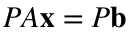.
We know that, so we can rewrite this as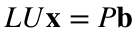.
If we rename, then we have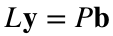.
This is a lower triangular system, so we can solve it with forward substitution to find. Once we have, we can use back substitution to solve, which gives us our final answer.
In MATLAB, the process looks like this:
[L, U, P] = lu(A);
y = L\(P*b);
x = U\y
x = 3×1
1 0 -1
• You must remember the permutation matrix P. MATLAB will allow the code [L, U] = lu(A), and you can even find the correct solution with y = L\b and x = U\y, but the matrices L and U will not be triangular, so this destroys the point of the process. In this class, if you are asked to use-decomposition, you have to explicitly find L, U and P, not just L and U.
• The parentheses on the second line are important. If you instead use y = L\P*b, you will get the same answer, but it will be substantially slower. (MATLAB does L\P first, which solves N different systems of equations, then puts all the solutions into a matrix and multiplies that matrix by b.)
• It is possible to combine the last two lines into one step with x = U\(L\(P*b)). As before, the parentheses are important. If you forget them, you will get the right answer but your code will run substantially more slowly. I will occasionally ask you for the intermediate vector y (either on a homework assignment or on a test), so you need to know how to do this in two steps.

### Speed of LU decomposition

The lu command uses essentially the same algorithm as Gaussian elimination, so we know that it takes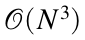flops. We then have to use forward substitution to solve, which takesflops, and then we have to use back substitution to solve, which takes anotherflops. The whole process therefore takes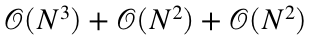flops, but since we only care about the largest power this means that it takesflops.
This is essentially the same speed as Gaussian elimination. (Which should make sense, since it's the same process, plus one more forward substitution step.) It therefore looks like we haven't actually made any improvements. The key thing to notice, though, is that the-decomposition step (i.e., finding the matrices L, U and P) only depends on A and not on. This means that if we have to solve two systems with the same left hand side, we only have to use the lu command once. For example, we can solve the systemwith the code
c = [4; 10; 24];
y = L\(P*c);
x = U\y
x = 3×1
1 1 1
Since we already have L, U and P, we don't have to use the lu command (which takesflops); we only have to use forward and back substitution (which both takeflops).
It turns out that this is an extremely common situation. Very often, the matrix A describes the permanent structure of a problem, while the right hand side of the system describes some temporary features. As an example, the left hand side might represent the location and orientation of different girders in a bridge, while the right hand side represents the loads from vehicles on the bridge. If we want to see how the bridge reacts to different traffic patterns, we will need to repeatedly solve linear systems with the same left hand side, but with different right hand sides. In such a situation, we can use the lu command once, and then solve all the other problems much more quickly.

### Inverses

There is one more solution method that you may see in textbooks or other classes. If you want to solve the system, then one possible approach is to multiply both sides of the equation by some matrix that will cancel out the A. Such a matrix is called the inverse of A and denoted by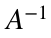. You would then solve the system by writing:, so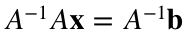, and so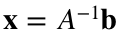.
We will essentially never compute an inverse matrix in this class, but MATLAB does have a command for it called inv. This means that you could solve the system by writing
x = inv(A) * b;
You should not use this code. The inv command is both slower and more prone to rounding error than Gaussian elimination. (This method is still technically, but it is worse than Gaussian elimination on every front.)
We will frequently use the notation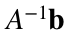in this class, but you should always mentally translate that into "the solution of the equation". Mathematically, they are the same thing, but in code you should never use inverses to solve a system.

### Summary of system solvers

We now know several different ways to solve a system of equations.
• If the system is lower/upper triangular, you can use forward/back substitution. The code for this in MATLAB is x = A\b. This process is.
• If you have to solve multiple systems with the same A, but different right hand sides, you can use-decomposition. The first system will takeflops, but subsequent systems will only takeflops.
• You can always fall back on Gaussian elimination. The code for this in MATLAB is also x = A\b, but if A is not triangular than it takesflops.
• You should not use matrix inverses.